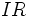# Ideal

This article gives a property that can be evaluated for a subset of a ring

## Definition

### Symbol-free definition

An ideal in a commutative unital ring (or any commutative ring) is a subset that satisfies the following equivalent conditions:

### Definition with symbols

An ideal in a commutative ring$R$ is a subset$I$ that satisfies the following equivalent conditions:

•$I$ an$R$-submodule of$R$.
•$I$ is an Abelian group under addition and further,$IR$ is contained inside$I$.

## Definition for noncommutative rings

For noncommutative rings, there are three notions:

## Property theory

### Intersection

An arbitrary intersection of ideals is again an ideal.

### Sum

The Abelian group generated by any family of ideals (when treated as Abelian groups) is itself an ideal, and is in fact the smallest ideal generated by them.

### Product

Further information: Product of ideals